Скачать презентацию NP-complete Problem 2 Prof S M Lee Department

d4bc48eec63c7fe8e5861d99a2a93595.ppt

• Количество слайдов: 29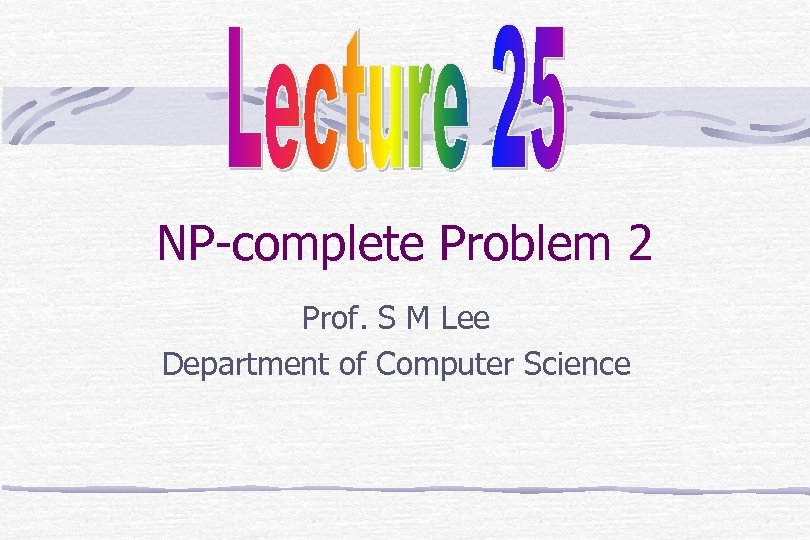NP-complete Problem 2 Prof. S M Lee Department of Computer Science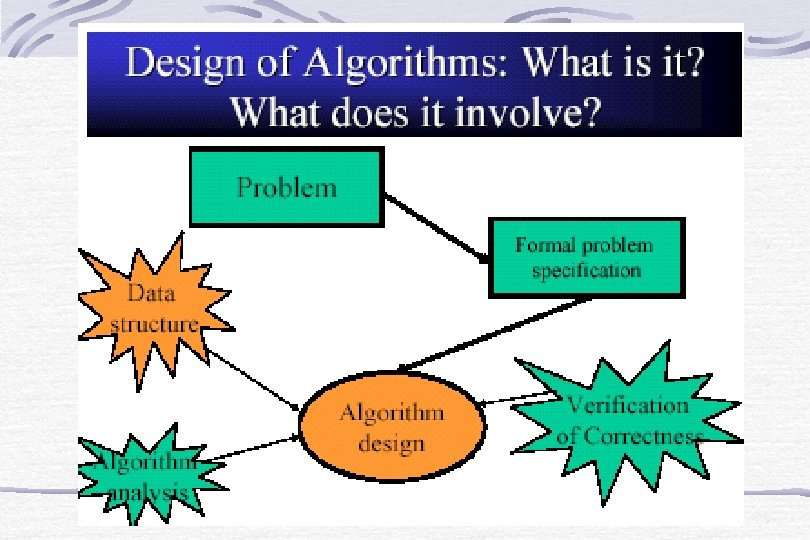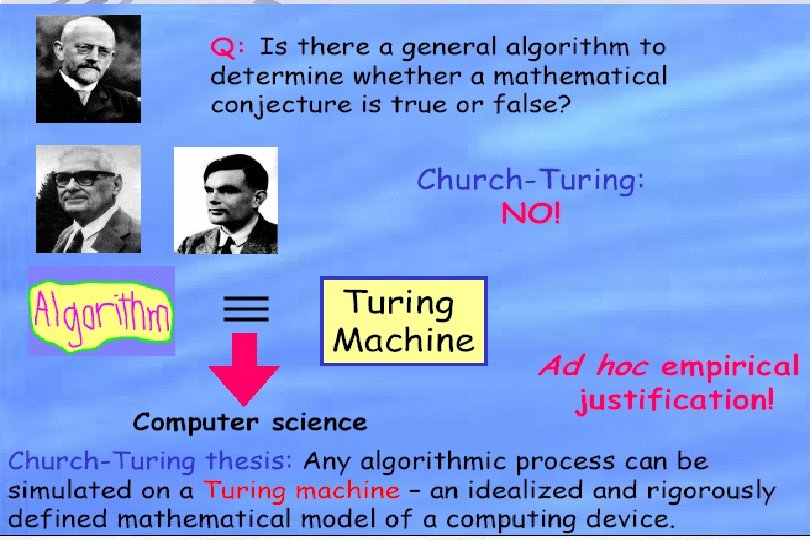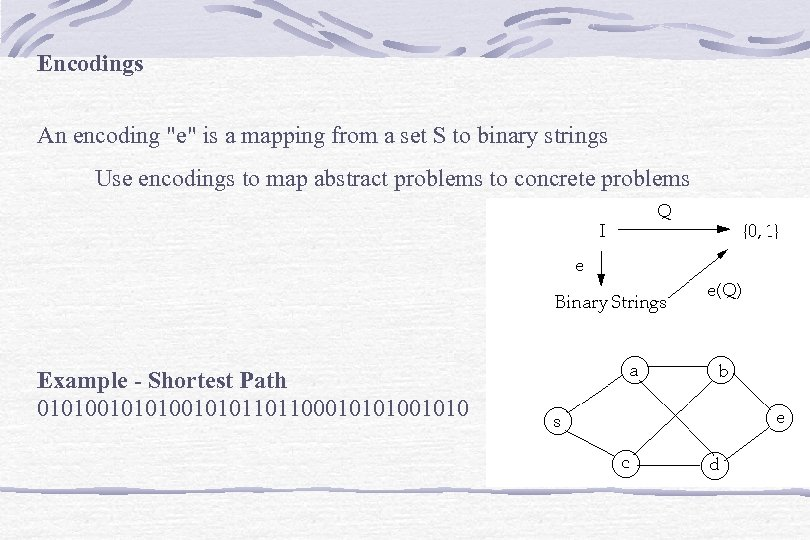Encodings An encoding "e" is a mapping from a set S to binary strings Use encodings to map abstract problems to concrete problems Example - Shortest Path 010100101011011000101010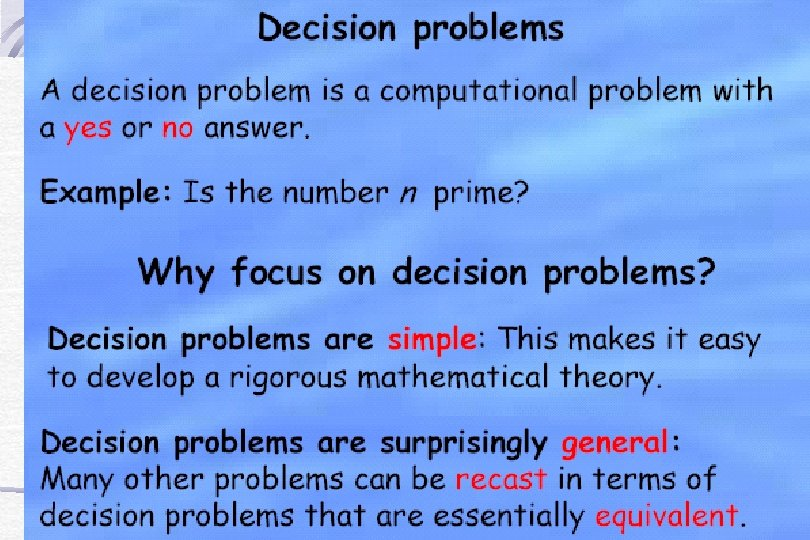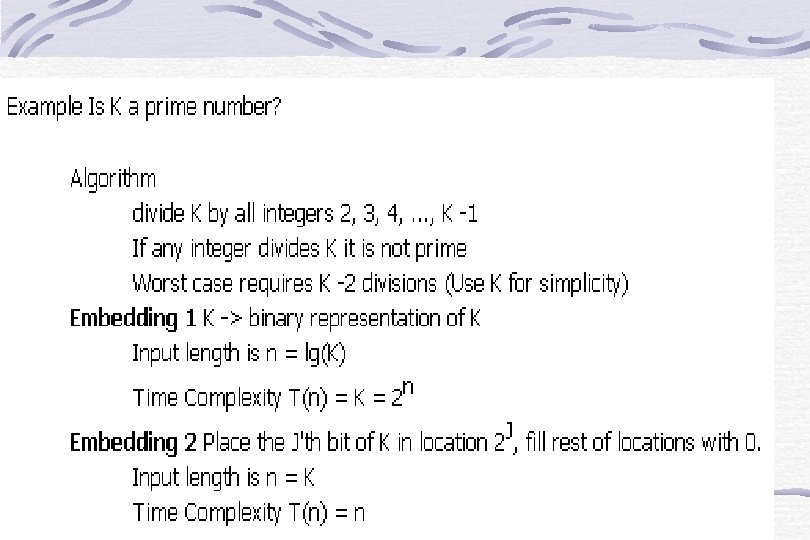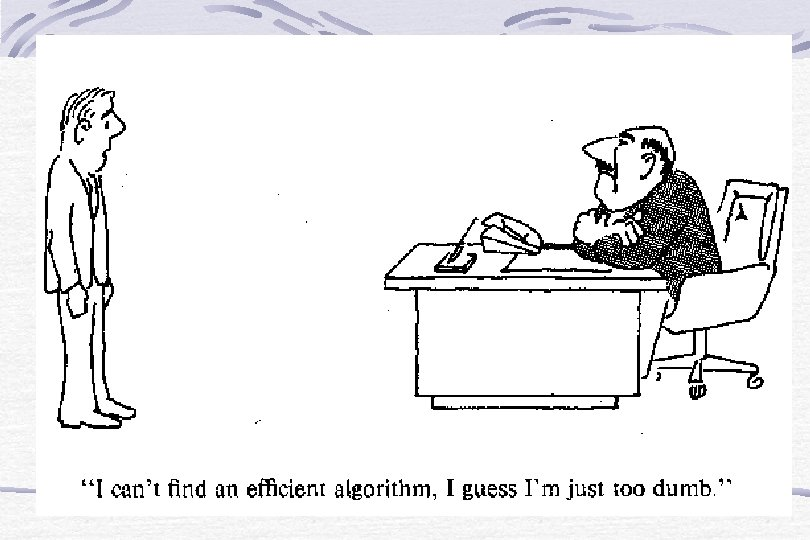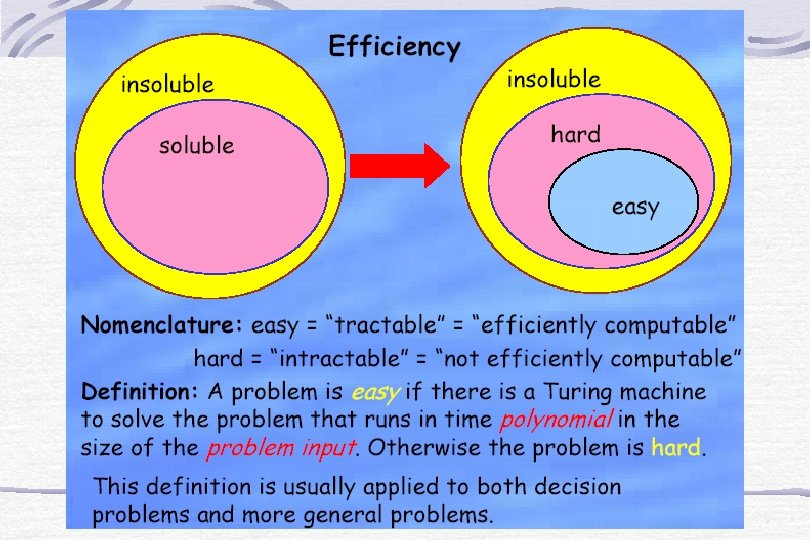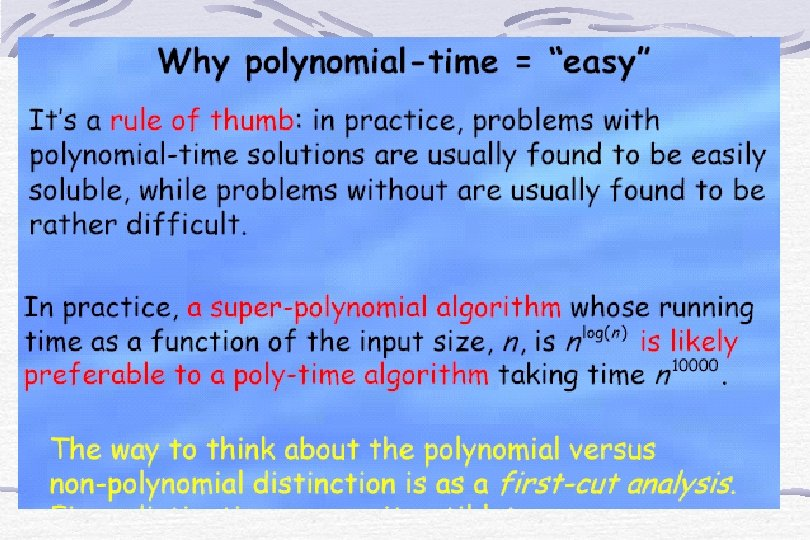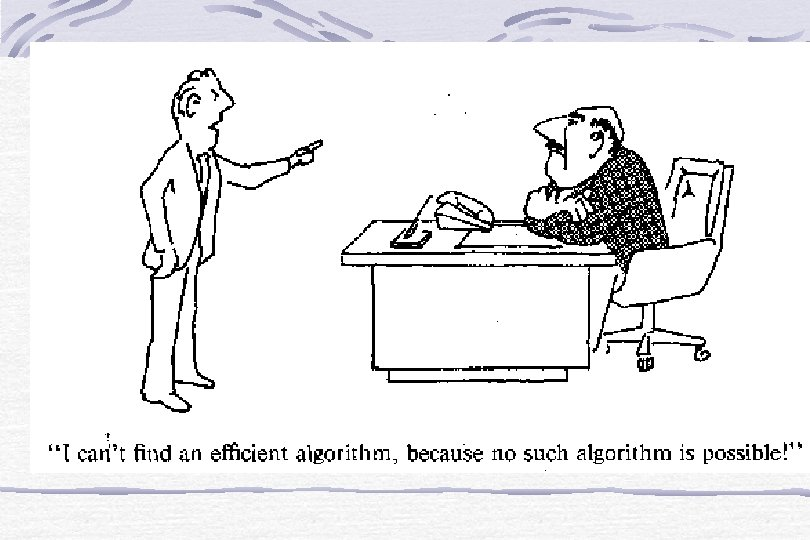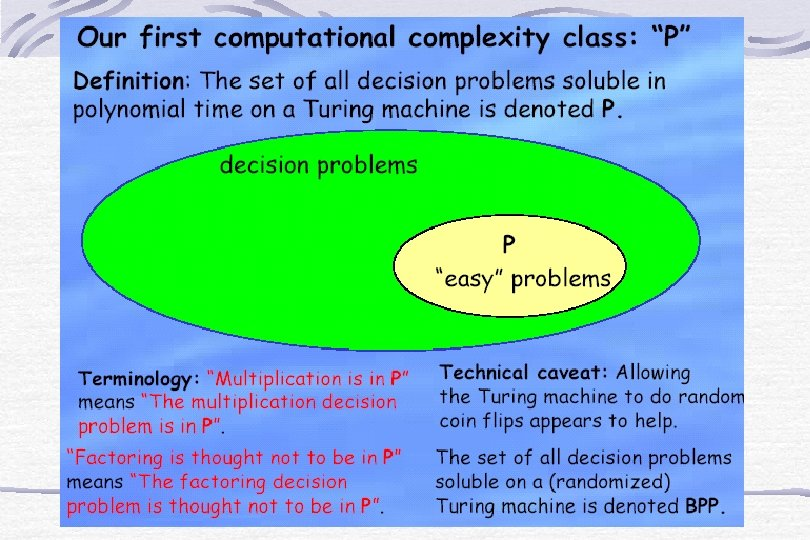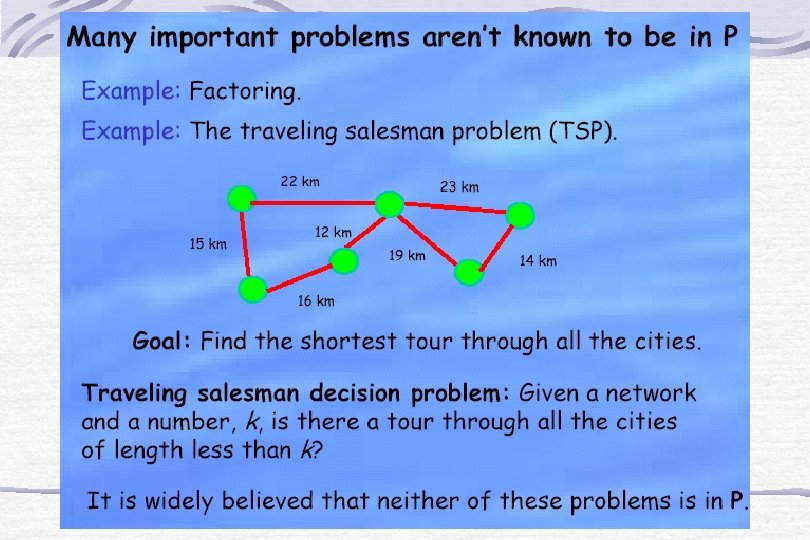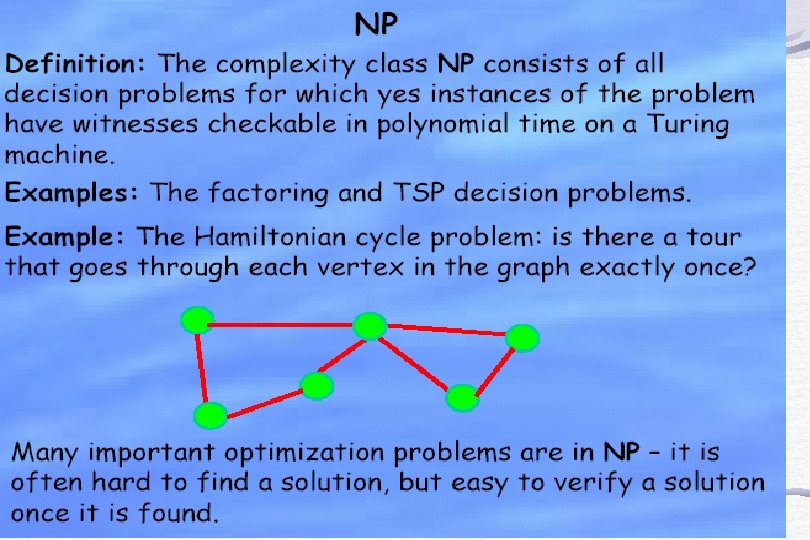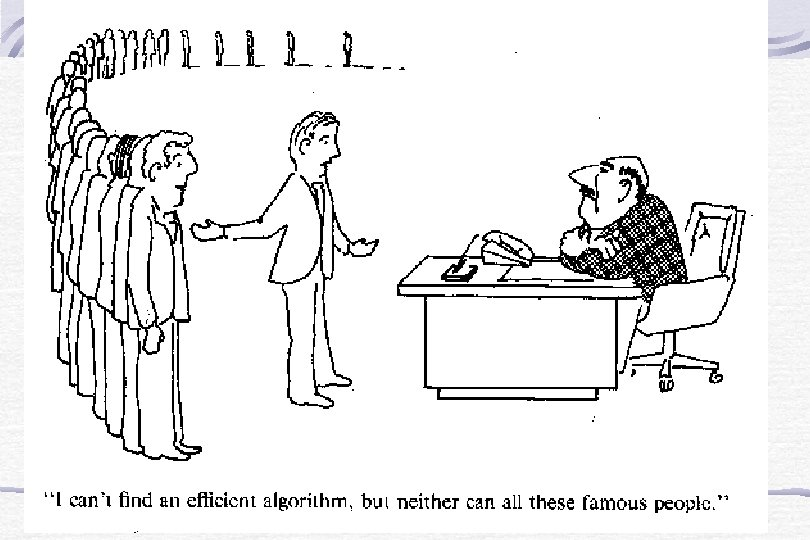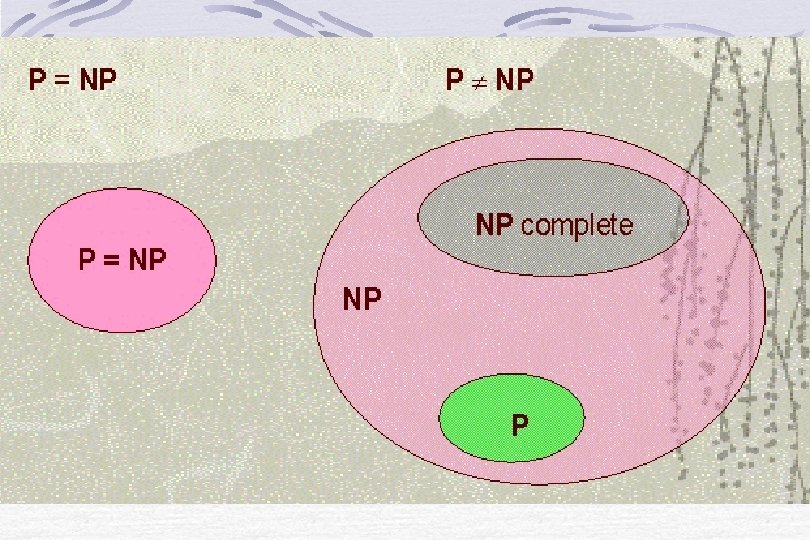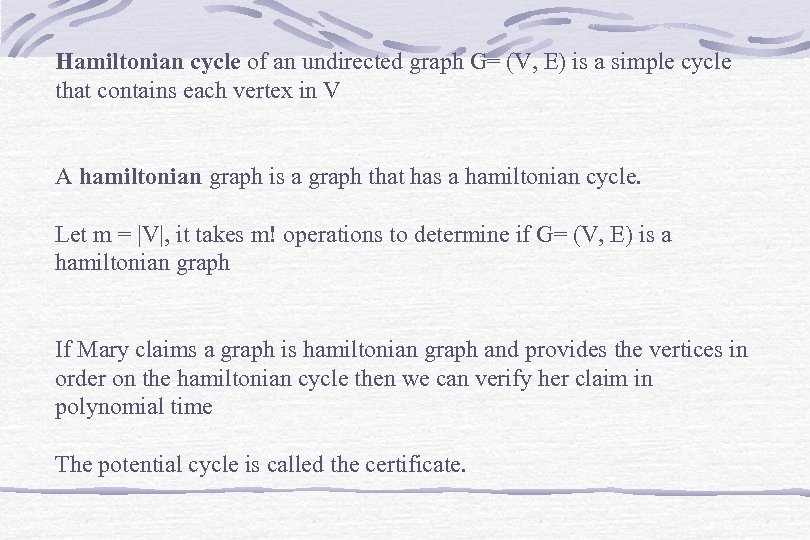Hamiltonian cycle of an undirected graph G= (V, E) is a simple cycle that contains each vertex in V A hamiltonian graph is a graph that has a hamiltonian cycle. Let m = |V|, it takes m! operations to determine if G= (V, E) is a hamiltonian graph If Mary claims a graph is hamiltonian graph and provides the vertices in order on the hamiltonian cycle then we can verify her claim in polynomial time The potential cycle is called the certificate.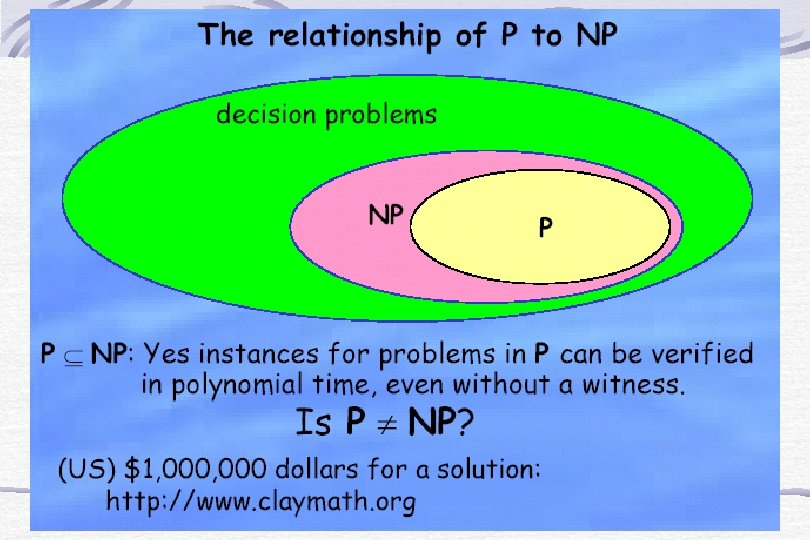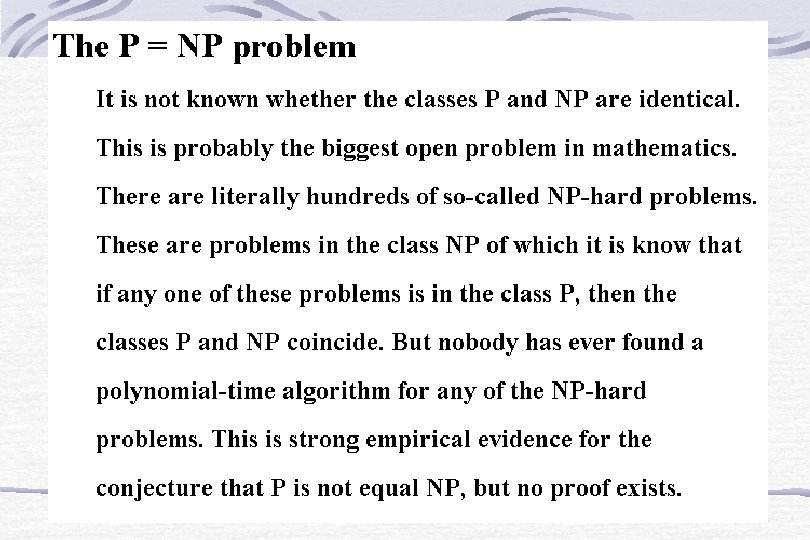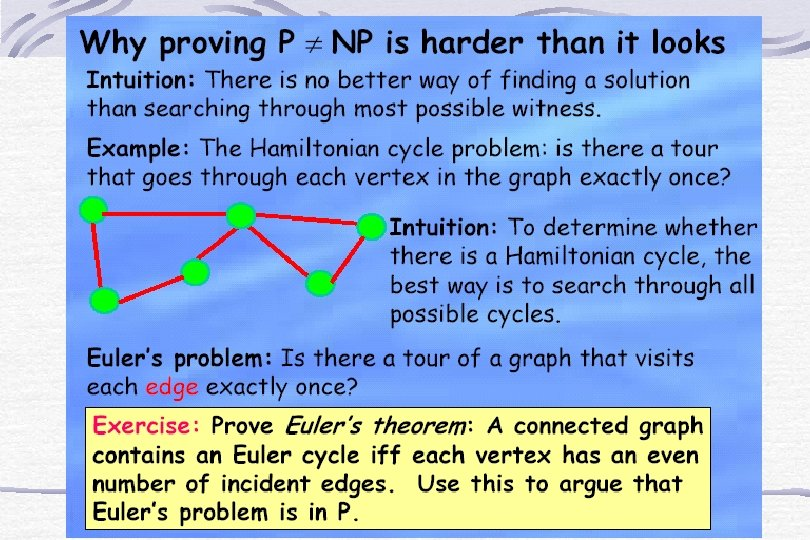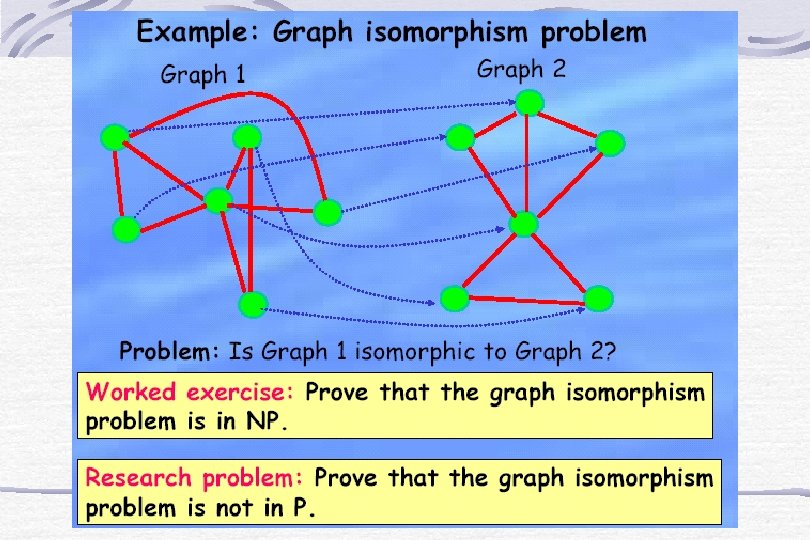Why Are We Studying NPcomplete Problems? Because we want to know what problems can be solved by computers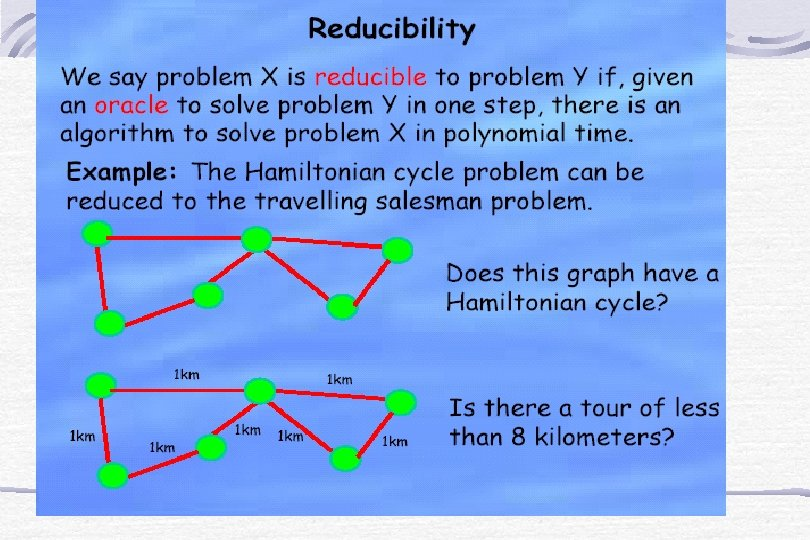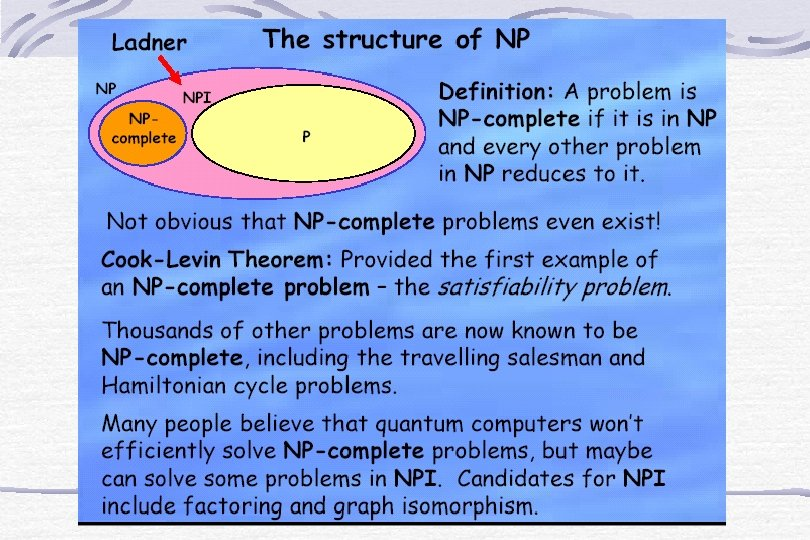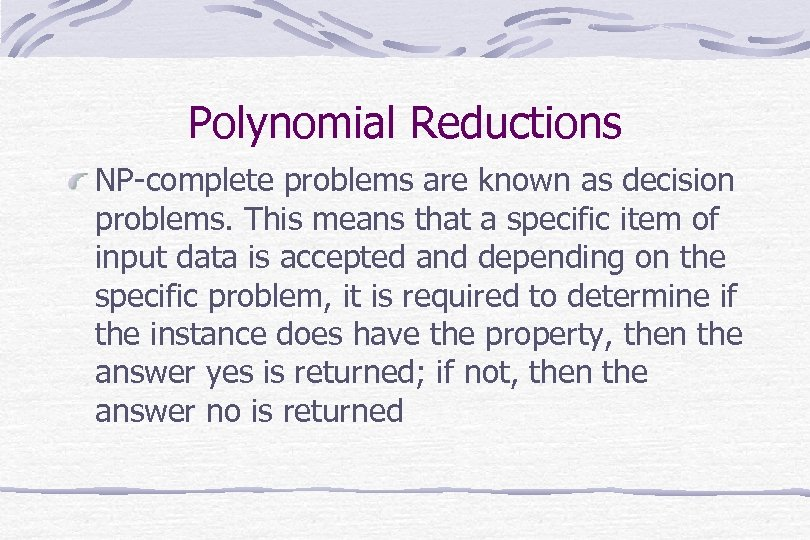Polynomial Reductions NP-complete problems are known as decision problems. This means that a specific item of input data is accepted and depending on the specific problem, it is required to determine if the instance does have the property, then the answer yes is returned; if not, then the answer no is returned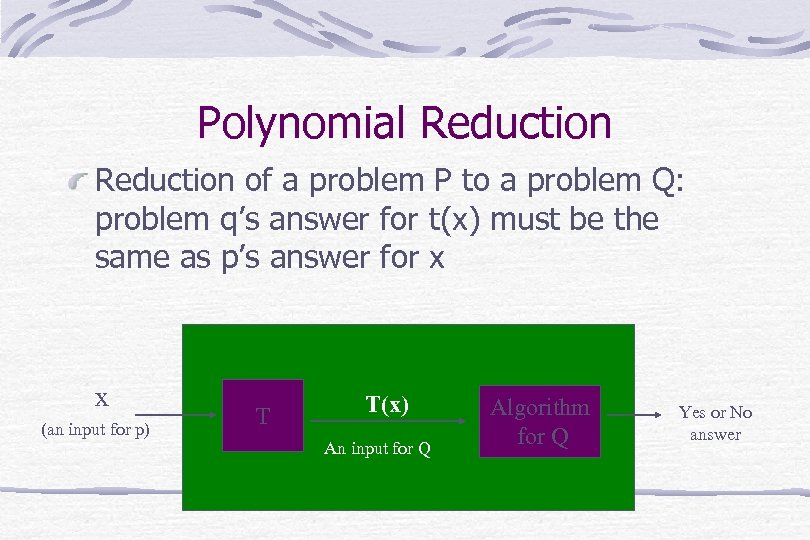Polynomial Reduction of a problem P to a problem Q: problem q’s answer for t(x) must be the same as p’s answer for x x (an input for p) T T(x) An input for Q Algorithm for Q Yes or No answer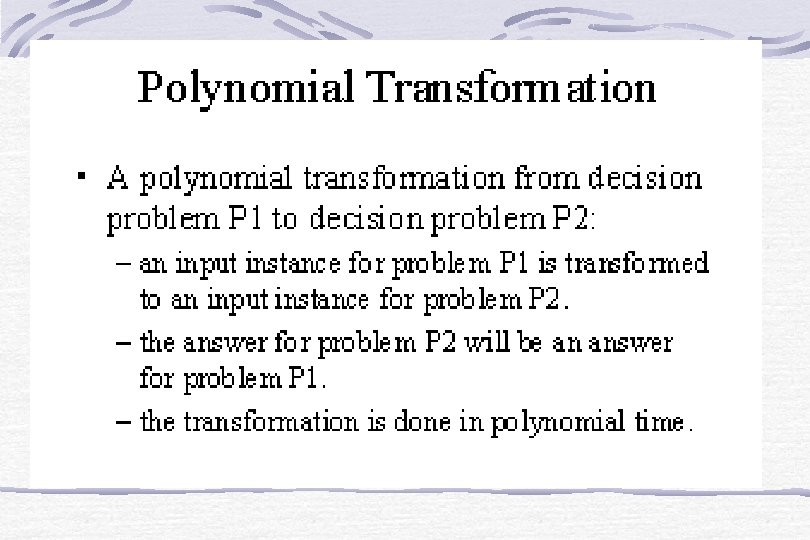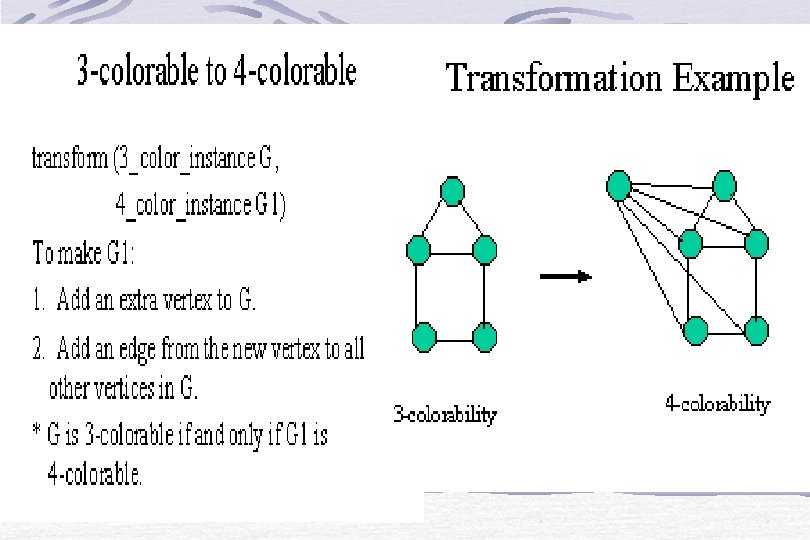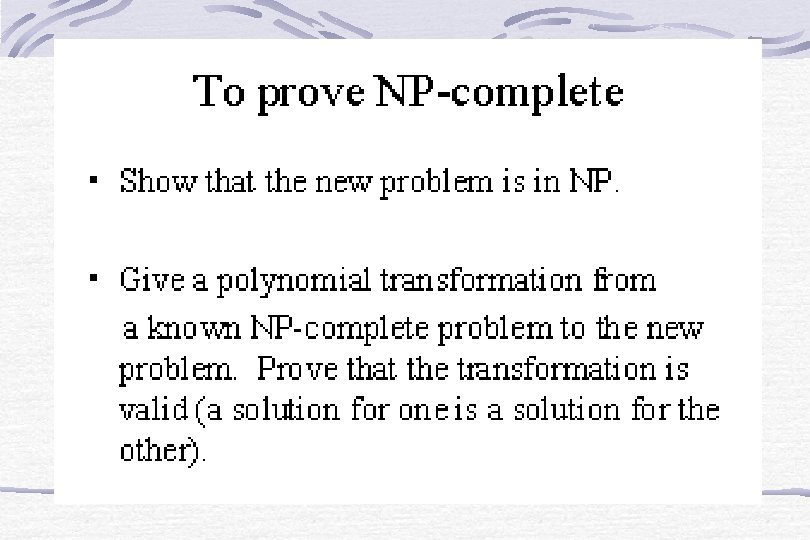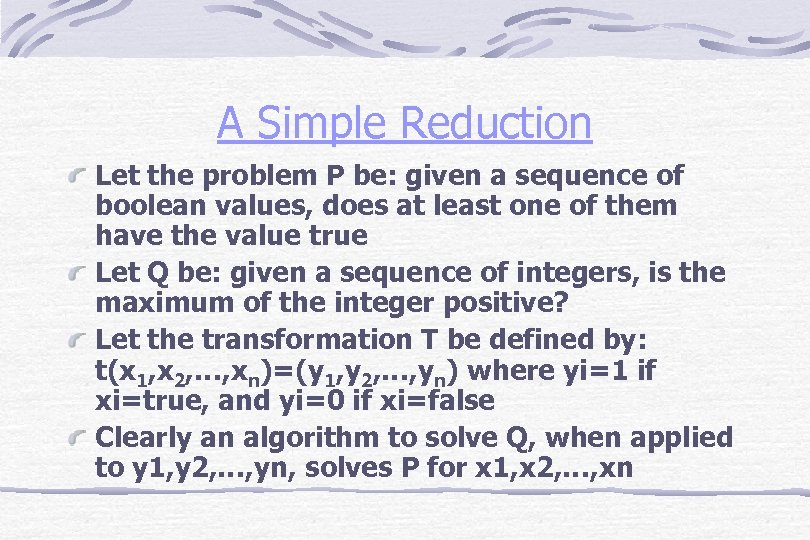A Simple Reduction Let the problem P be: given a sequence of boolean values, does at least one of them have the value true Let Q be: given a sequence of integers, is the maximum of the integer positive? Let the transformation T be defined by: t(x 1, x 2, …, xn)=(y 1, y 2, …, yn) where yi=1 if xi=true, and yi=0 if xi=false Clearly an algorithm to solve Q, when applied to y 1, y 2, …, yn, solves P for x 1, x 2, …, xn